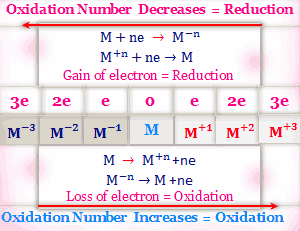# Oxidation reduction reactions

### Oxidation number and concept

The oxidation number of CH4, CH3Cl, CH2Cl2, CHCl3, CCl4 and CO2 are -4, -2, 0, +2, +4 and +4 respectively. In H2, the oxidation number of hydrogen is zero but in H2O it is +1. Similarly, magnesium in the elementary state has a zero oxidation number but in MgCl2, the oxidation number is +2.
From the above, we can define Oxidation and Reduction according to the Oxidation number increase or decrees,

#### Oxidation

Oxidation may be defined as a process in which an increase in oxidation number by atoms or ions occurs. A reagent which can increase the oxidation number of an element or ion is called an oxidizing agent.

#### Reduction

The reduction is a process in which a decrease in oxidation number by atoms or ions occurs. A reagent that lowers the oxidation number of an element or ion is called a reducing agent.

#### Representation of oxidation and reduction

Oxidation and reduction are always found to go hand to hand during a redox reaction. Whenever an element or a compound is oxidized, another element or another compound must be simultaneously reduced.
An oxidant is reduced and simultaneously the reductant is oxidized.Oxidation number and oxidation-reduction
H2S + Cl2 → 2HCl + S
1. Magnesium metal burns in oxygen to produce magnesium oxide: Before the reaction oxidation number of magnesium and oxygen both zero. But after the reaction, the oxidation number of magnesium and oxygen is +2 and -2 respectively. Thus the oxidation number of magnesium is increases and the oxidation number of oxygen is decreased. So magnesium is oxidized and oxygen is reduced.
Mg + O2 → 2MgO
2. The reaction between Sodium and Chlorine: From the same way, we can explain this reaction. Here oxidation number of Sodium and Chlorine is zero before the reaction and +1 and -1 after the reaction. Thus sodium oxidized and chlorine reduced.
Na + Cl2 → 2NaCl
Problem
Why sulfur dioxide has properties of oxidation and reduction?
This can be explained by the oxidation state of sulfur. The oxidation numbers of sulfur in the compounds H2S, SO2, and SO3 are -2, +4 and +6 respectively. Thus the highest oxidation state of sulfur is +6 and the lowest is -2.
The oxidation state of the free sulfur element is 0. In SO2, the oxidation state of sulfur is +4, this oxidation state is the middle of 0 and +6.
Thus, the oxidation state can increases from +4 to +6 and decreases from +4 to 0.

SO₂ + H₂S → 2H₂O + 3S